Searching...

# Tech Tips

### Acceleration Voltage of Electron Microscope

The electron microscope is an indispensable instrument for analyzing samples. This series explains the basics of electron microscopes. The first theme is the acceleration voltage of the electron microscope.
An electron microscope is a device that uses an electron beam to obtain an enlarged image of an observation object (hereinafter referred to as a sample). An electron beam is a lot of electrons that are converged and emitted in one direction. When an electron beam is viewed as a wave, the wavelength is very short. Therefore, it is possible to observe at much higher magnification (more precisely speaking, higher resolution) than optical microscopes.
In an electron microscope, electrons are accelerated by applying a high voltage emitted from the electron gun, converged by a focusing lens (converging coil) using an electromagnet, and then applied to the object to be observed. In the case of a transmission electron microscope (TEM), when the sample is made thin, the electron beam will be transmitted. If this transmitted electron beam is guided to the fluorescent screen via the objective lens, intermediate lens, and magnifying lens, the brightness of the fluorescent screen varies depending on the number of transmitted electrons. This can be imaged by shooting with a camera.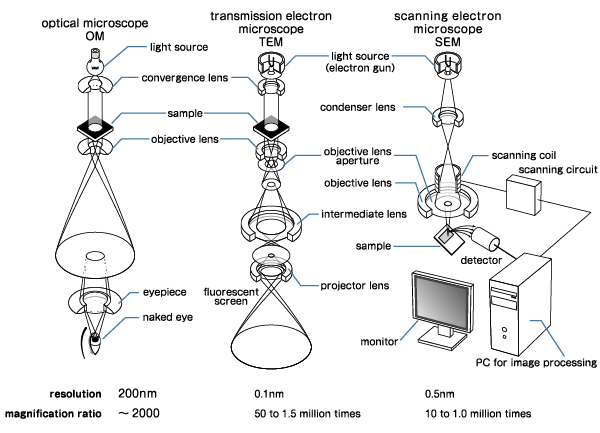In the case of a scanning electron microscope (SEM), an electron beam is applied to the sample via a focusing coil, a scanning coil, and an objective lens. By narrowing down the electron beam as much as possible and scanning the surface, the secondary electron generated according to the shape of the sample hit by the electron beam is observed with a detector to obtain an image.
How can I increase the resolution of the electron microscope? Unlike the optical microscope, the following formula for the resolution is used for the electron microscope (TEM).

d = 0.65 (Csλ3) 1/4

d is the resolution, Cs is the spherical aberration coefficient, and λ is the wavelength of the electron beam. From this formula, to increase the resolution, in other words, to decrease the value of d, either decrease Cs or decrease λ. However, since it is difficult to improve Cs with an electron lens such as a focusing lens used in an electron microscope, the resolution is generally increased by reducing the wavelength λ.
The relationship between the wavelength λ of the electron beam and the acceleration voltage V is expressed by the following equation.

λ = 1.23/V1/2 (nm)

For example, if the electron acceleration voltage is 100 kV, the wavelength is 3.9 x 10-3 nm. If Cs = 0.5 mm, the resolution d is as follows.

• d = 0.65 {0.5 x 106 x (3.9 x 10-3)3}1/4
• = 0.65 {2.97 x 10-2}1/4
• = 0.27

Therefore, if the acceleration voltage is increased to 400 kV, the resolution is calculated to be less than 0.1 nm. Although the calculation formula is different in SEM, the resolution is basically improved by increasing the acceleration voltage.
However, there are problems with increasing the acceleration voltage. First, due to Einstein's special theory of relativity, increasing the acceleration voltage will increase the mass of electrons. For that reason, increasing the energy for acceleration does not increase the speed and the wavelength does not shorten.
The resolution will also be affected. For example, in the case of SEM, secondary electrons due to reflected electrons are generated in addition to secondary electrons due to incident electrons. When the acceleration voltage is increased, the electrons are reflected after reaching a deeper position on the sample, and the reflected electrons come away from the incident position, making it impossible to examine the fine structure of the surface.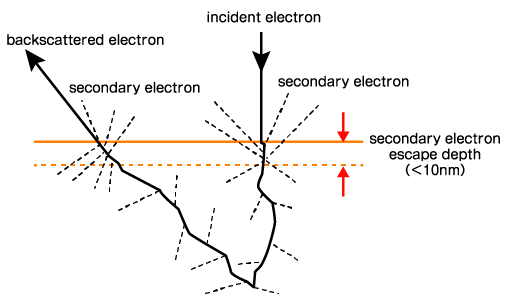In addition, since the energy of electrons increases when the acceleration voltage is high, when the sample is an insulator, problems such as surface charging will occur. In order to avoid this in SEM, when using an insulating material as a sample, a pretreatment is required to apply a coating by depositing a conductor.
These problems are the result of efforts to shorten the wavelength of electron beams in order to increase resolution in Japan. On the other hand, in Germany, efforts have been made to improve the spherical aberration coefficient. As a result, the resolution can be improved even with the same acceleration voltage.
In addition, SEM also employs a method in which the acceleration voltage is lowered to prevent the insulator surface from being charged.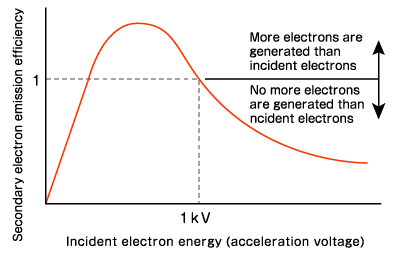The efficiency of secondary electron emission decreases as the acceleration voltage exceeds 1 kV. The part where the emission efficiency is lowered is used for charging the insulator, but no charge occurs when the secondary electron emission efficiency is just around 1. Therefore, SEM eliminates the need for coating as a pretreatment if the acceleration voltage is used.

However, simply reducing the acceleration voltage is not enough. Lowering the acceleration voltage means increasing the wavelength of the electron beam, so the resolution will inevitably decrease. In particular, the resolution rapidly drops below a few kV. This is because aberration occurs due to variations in the energy of electrons emitted from the electron gun.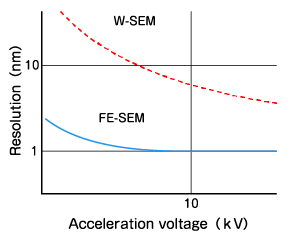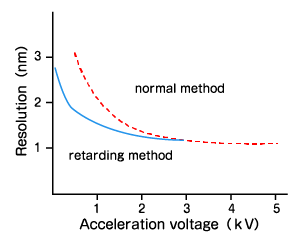But there is a way around this problem. That is the "retarding method". Electrons with little variation are emitted from the electron gun at a high acceleration, and an electric field for decelerating the electrons at the objective lens is generated just before hitting the sample. This method reduces the acceleration voltage that collides with the sample while keeping the aberration small.
In this case, since aberrations are reduced, there is an advantage that higher resolution can be maintained than when observing at normal low acceleration voltage. Of course, it will be necessary to add a circuit to the objective lens, so the structure itself will be a little complicated.
At this time, a method in which a circuit is added to the objective lens to decelerate the electrons while passing through the objective lens is called an "immersion lens method". On the other hand, a method in which the electron is decelerated before it collides with the sample after passing through the objective lens is called a "cathode lens method".

In the immersion lens method, the electric field near the sample is weakened, so the surface shape of the sample does not easily affect the lens characteristics. On the other hand, the cathode lens method has the advantage that higher resolution can be obtained by increasing the deceleration electric field.
Then, how should we set the optimum accelerating voltage according to the material of the sample based on these? In the case of TEM, the correct answer is to consider how fine you want to see the structure and apply an acceleration voltage to obtain the desired resolution.
On the other hand, in the case of SEM, the correct answer is to change it depending on the elements contained in the sample. In other words, when the surface structure is examined, you need to consider the element contained in the surface and the electron orbit of element to be excited by the electron beam.

For example, in the case of Fe, Kα (6.40 keV) and Kβ1 (7.06 keV) will be excited if the acceleration voltage is 7.11 kV or higher. On the other hand, Lα (0.70 keV) are excited when 0.71 kV or higher, so a high acceleration voltage is not necessary. In order to measure with good sensitivity while increasing the spatial resolution, it is only necessary to select the elements to be measured in the sample with low minimum excitation energy and apply an acceleration voltage that excites them with sufficient intensity.

Next time, as an electron microscope (SEM) technical explanation series (2), we will introduce the electron microscope lens.

### Recommended products

Matsusada Precision manufactures precision power supplies for scanning electron microscopes. That is multi-channel power supplies for electron guns, high-voltage modular power supplies for secondary detectors, and bipolar power supplies for magnetic lenses in SEM.